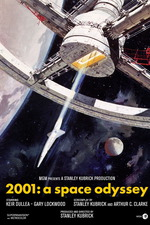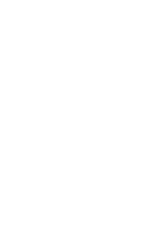2001: A Space Odyssey

## 2001: A Space Odyssey 1968

8 A.M. Sunday, 12th of January, 2014. HAL'S birthday. I'm tired as fuck.

HAL.

IBM.

HAL/IBM. HAL=IBM. IBM=HAL.

H+1 = I
A+1 = B
L+1 = M

So HAL is indisputably IBM. Just go one letter on in the alphabet from H, A and L and you get I, B and M.

But what is IBM? A computer company Kubrick had a grudge against? Or are we just missing a "T" in the greatest riddle known to mankind? Confused? Allow me to demonstrate.

IBM
I= 9th letter of the alphabet.
B= 2nd letter of the alphabet.
M= 13th letter of the alphabet.

9+2+13= 24.

X= 24th letter of the alphabet.

X= 10 in Roman numerals, so IBM= 10.

HAL
H= 8th letter of the alphabet.
A= 1st letter of the alphabet.
L= 12th letter of the alphabet.

8+1+12= 21.

Separate that answer, what do you have? 2  1.

2x1=2, so HAL= 2

Now to get our "T". So if IBM= X, or 10 and HAL= 2 then we should combine the two like so:

10x2= 20.

So HAL x IBM= 20.

T= 20th letter of the alphabet.

We have now found our "T", but what is its significance you ask? The fact that "T" is the hidden letter in the HAL/IBM conundrum and the fact that IBM is hidden in HAL brings us to the conclusion that IBM and T should be combined. But where to put the "T"?

IBM or InBetween Me. So, IBTM.

And now for the big reveal.

I
B
T
M

Or

I
Built
The
Moon

Thus proving once and for all that the moon landing footage was in fact faked by Mr Kubrick. Someone find out if those Room 237 guys want to interview me.

(I broke some rules, Lise. I'm sorry)

All